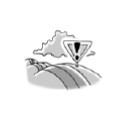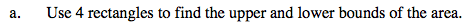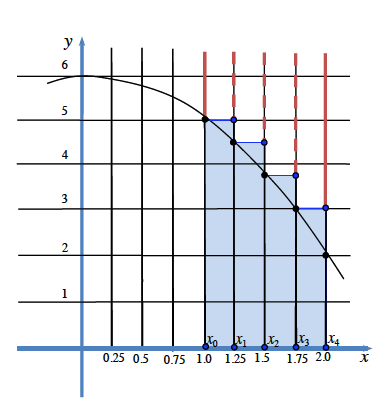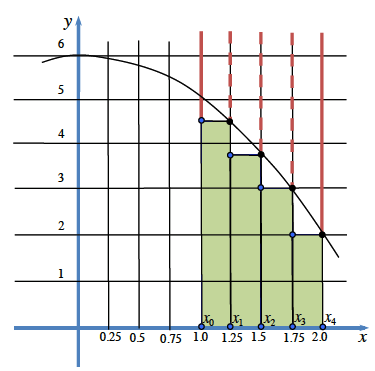### Home > PC > Chapter 2 > Lesson 2.3.5 > Problem2-132

2-132.
1. You are trying to find the area A(f, 1 ≤ x ≤ 2) for f(x) = −x2 + 6. Homework Help ✎1. Use 4 rectangles to find the upper and lower bounds of the area.

2. Express the sum of the lower bound using sigma notation.Upper Bound means that the rectangles will give an over estimate.Lower Bound means that the rectangles will give an under estimate.$.25\sum_{k=1}^{4}-(\text{find this expression})^{2}+6$